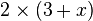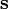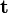# Term (mathematics) facts for kids

Kids Encyclopedia Facts

A term is a mathematical expression.

## Definition

In elementary mathematics, a term is either a single number or variable, or the product of several numbers or variables. Terms are separated by a + or - sign in an overall expression. For example, in

3 + 4x + 5yzw

3, 4x, and 5yzw are three separate terms.

In the context of polynomials, term can mean a monomial with a coefficient. To combine like terms in a polynomial is to make it a linear combination of distinct monomials. For example,

3x + 2x2 + 5x + 1 = 2x2 + (3+5)x + 1 = 2x2 + 8x + 1, with like terms collected.

A series is often represented as the sum of a sequence of terms.

In general mathematical use, however, term is not limited to additive expressions. Individual factors in an expression representing a product are multiplicative terms. Indeed, individual elements of any mathematical expression may be referred to as "terms".

In logic, a term is a collection of symbols used to represent a mathematical object (rather than a mathematical statement). A basic term is either a constant or a variable, while more complex terms can also be built using operators (such as the term$2 \times (3 + x)$). To refer to a term, variables such as$\mathbf{s}$ and$\mathbf{t}$ are often used.

In informal logic, a term can also refer to the subject or predicate of a statement.

## Specific names for terms